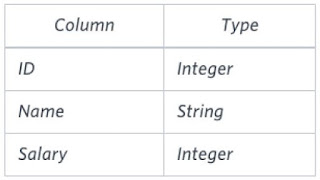# HackerRank: [SQL Aggregation] (7/17) THE BLUNDER | ceil, avg & replace function in SQL

I started studying SQL from a very famous site - HackerRank. Here I will try to provide multiple approaches & solutions to the same problem. It will help you learn and understand SQL in a better way.

Please make use of my blog posts for learning purpose only and feel free to ask your questions in the comment box below in case of any doubt.

SQL Problem Statement:

Samantha was tasked with calculating the average monthly salaries for all employees in the EMPLOYEES table, but did not realize her keyboard's 0 key was broken until after completing the calculation. She wants your help finding the difference between her miscalculation (using salaries with any zeroes removed),and the actual average salary.

Write a query calculating the amount of error (i.e.: actual - miscalculated average monthly salaries), and round it up to the next integer.

Input Format:

The EMPLOYEES table is described as follows:Table: EMPLOYEES Columns

Note:
Salary is measured in dollars per month and its value is 10^5.

Sample Input:
The table below shows the salaries without zeroes as they were entered by Samantha:

Samantha computes an average salary of 98.00. The actual average salary is 2159.00.
The resulting error between the two calculations is 2159.00 - 98.00 = 2061.00 which, when rounded to the next integer, is 2061.

### Solution: Using MAX & MIN Function (MySQL Query):

```SELECT CEIL(AVG(SALARY) - AVG(REPLACE(SALARY, '0', '')))
FROM EMPLOYEES;```

NOTE:
1. AVG function used to get the average of the values of all the records in the specified column name passed to the function.
2. CEIL function used to get the nearest integer of the original number passed to the function. (The output will be always greater than or equal to the original number).
Eg. CEIL(2.34) will return 3
3. REPLACE function is used to replace all occurrences of the substring within an input string with another substring. Here, '0' is replaced with a blank ('') character.